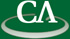## Math on the Web: from posting assignments to mathematical web services.

### Stephen Watt, University of Western Ontario

```How will mathematics be represented on the World Wide Web?
Why not just use TeX?

This talk examines the consequences of using different means to
represent mathematical expressions in Web documents and digital
libraries.  We describe MathML, an XML application devised by the
World Wide Web Consortium, and OpenMath, an extensible protocol
used in a number of European projects.

The talk considers the exchange of mathematical expressions via
the web both between people and machines:  We begin with a view
of people composing and sharing mathematical documents and then
explore protocols for machines to cooperate in providing
mathematical services.
```